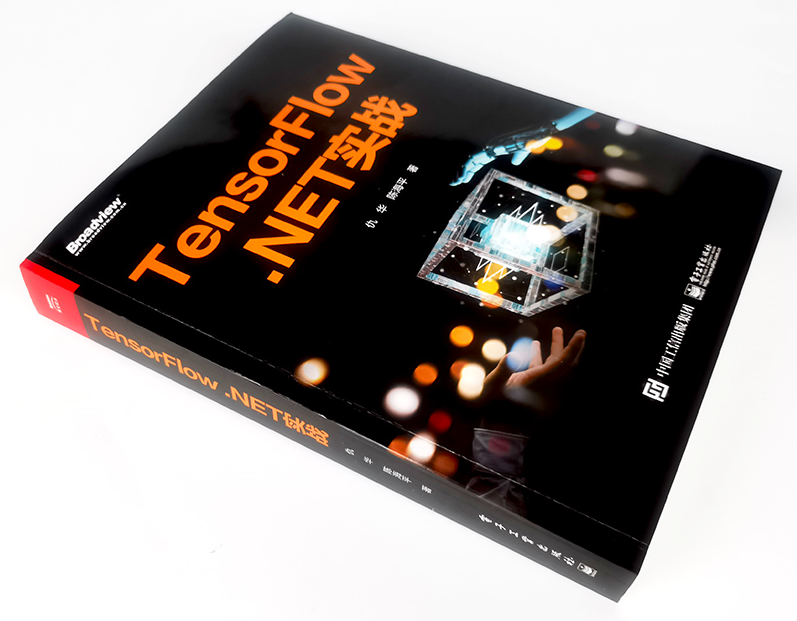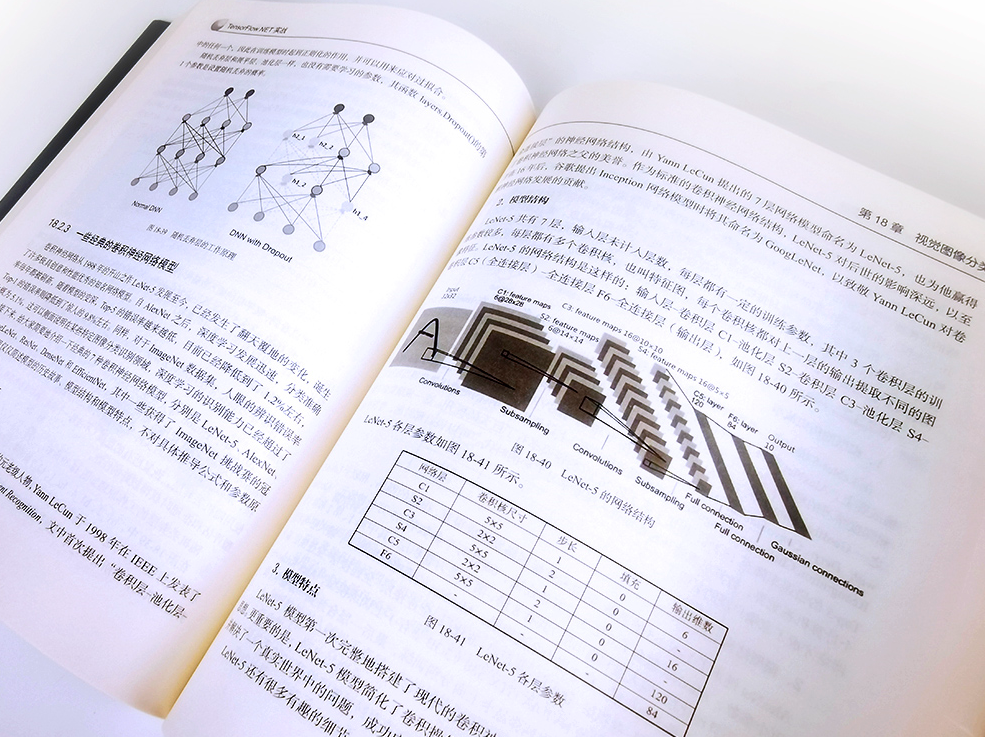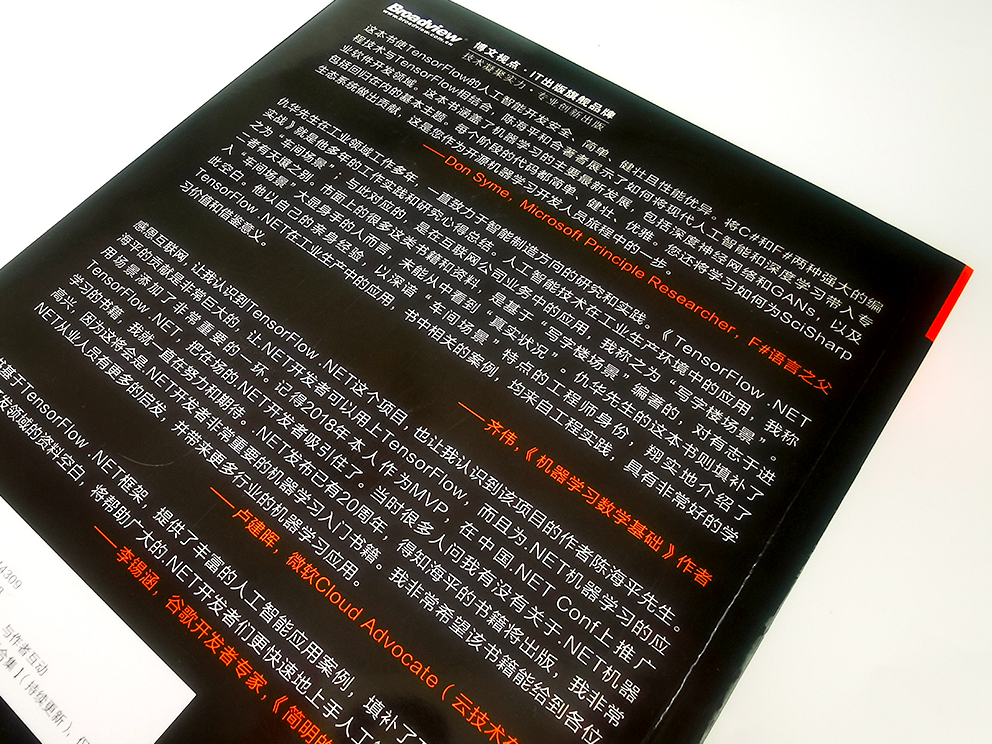You can not select more than 25 topics Topics must start with a letter or number, can include dashes ('-') and can be up to 35 characters long.Rinne 6fb930aa6dTensorFlow.NET (TF.NET) provides a .NET Standard binding for TensorFlow. It aims to implement the complete Tensorflow API in C# which allows .NET developers to develop, train and deploy Machine Learning models with the cross-platform .NET Standard framework. TensorFlow.NET has built-in Keras high-level interface and is released as an independent package TensorFlow.Keras.

English | 中文

=========================================================

### Voting: Naming Convention Approach of v1.0.0

Dear all,

We would like to urge you to participate in our upcoming vote regarding the naming convention for TensorFlow.NET version 1.0.0 in #1074. Your participation in the vote is essential to help us decide on the best approach for improving the naming convention used in previous versions.

Thank you,

TensorFlow.NET Authors

=========================================================

master branch and v0.100.x is corresponding to tensorflow v2.10, v0.6x branch is from tensorflow v2.6, v0.15-tensorflow1.15 is from tensorflow1.15. Please add `https://www.myget.org/F/scisharp/api/v3/index.json` to nuget source to use nightly release.## Why Tensorflow.NET ?

`SciSharp STACK`'s mission is to bring popular data science technology into the .NET world and to provide .NET developers with a powerful Machine Learning tool set without reinventing the wheel. Since the APIs are kept as similar as possible you can immediately adapt any existing TensorFlow code in C# or F# with a zero learning curve. Take a look at a comparison picture and see how comfortably a TensorFlow/Python script translates into a C# program with TensorFlow.NET.SciSharp's philosophy allows a large number of machine learning code written in Python to be quickly migrated to .NET, enabling .NET developers to use cutting edge machine learning models and access a vast number of TensorFlow resources which would not be possible without this project.

In comparison to other projects, like for instance TensorFlowSharp which only provide TensorFlow's low-level C++ API and can only run models that were built using Python, Tensorflow.NET makes it possible to build the pipeline of training and inference with pure C# and F#. Besides, Tensorflow.NET provides binding of Tensorflow.Keras to make it easy to transfer your code from python to .NET.

ML.NET also take Tensorflow.NET as one of the backends to train and infer your model, which provides better integration with .NET.

## Documention

Introduction and simple examplesTensorflow.NET Documents

Detailed documentionThe Definitive Guide to Tensorflow.NET

ExamplesTensorFlow.NET Examples

Troubleshooting of running example or installationTensorflow.NET FAQ

## Usage

### Installation

You can search the package name in NuGet Manager, or use the commands below in package manager console.

The installation contains two parts, the first is the main body:

``````### Install Tensorflow.NET
PM> Install-Package TensorFlow.NET

### Install Tensorflow.Keras
PM> Install-Package TensorFlow.Keras
``````

The second part is the computing support part. Only one of the following packages is needed, depending on your device and system.

``````### CPU version for Windows, Linux and Mac
PM> Install-Package SciSharp.TensorFlow.Redist

### GPU version for Windows (CUDA and cuDNN are required)
PM> Install-Package SciSharp.TensorFlow.Redist-Windows-GPU

### GPU version for Linux (CUDA and cuDNN are required)
PM> Install-Package SciSharp.TensorFlow.Redist-Linux-GPU
``````

Two simple examples are given here to introduce the basic usage of Tensorflow.NET. As you can see, it's easy to write C# code just like that in Python.

### Example - Linear Regression in `Eager` mode

``````using static Tensorflow.Binding;
using static Tensorflow.KerasApi;
using Tensorflow;
using Tensorflow.NumPy;

// Parameters
var training_steps = 1000;
var learning_rate = 0.01f;
var display_step = 100;

// Sample data
var X = np.array(3.3f, 4.4f, 5.5f, 6.71f, 6.93f, 4.168f, 9.779f, 6.182f, 7.59f, 2.167f,
7.042f, 10.791f, 5.313f, 7.997f, 5.654f, 9.27f, 3.1f);
var Y = np.array(1.7f, 2.76f, 2.09f, 3.19f, 1.694f, 1.573f, 3.366f, 2.596f, 2.53f, 1.221f,
2.827f, 3.465f, 1.65f, 2.904f, 2.42f, 2.94f, 1.3f);
var n_samples = X.shape;

// We can set a fixed init value in order to demo
var W = tf.Variable(-0.06f, name: "weight");
var b = tf.Variable(-0.73f, name: "bias");
var optimizer = keras.optimizers.SGD(learning_rate);

// Run training for the given number of steps.
foreach (var step in range(1, training_steps + 1))
{
// Run the optimization to update W and b values.
// Wrap computation inside a GradientTape for automatic differentiation.
// Linear regression (Wx + b).
var pred = W * X + b;
// Mean square error.
var loss = tf.reduce_sum(tf.pow(pred - Y, 2)) / (2 * n_samples);
// should stop recording

// Update W and b following gradients.

if (step % display_step == 0)
{
pred = W * X + b;
loss = tf.reduce_sum(tf.pow(pred - Y, 2)) / (2 * n_samples);
print(\$"step: {step}, loss: {loss.numpy()}, W: {W.numpy()}, b: {b.numpy()}");
}
}
``````

Run this example in Jupyter Notebook.

### Example - Toy version of `ResNet` in `Keras` functional API

``````using static Tensorflow.Binding;
using static Tensorflow.KerasApi;
using Tensorflow;
using Tensorflow.NumPy;

var layers = keras.layers;
// input layer
var inputs = keras.Input(shape: (32, 32, 3), name: "img");
// convolutional layer
var x = layers.Conv2D(32, 3, activation: "relu").Apply(inputs);
x = layers.Conv2D(64, 3, activation: "relu").Apply(x);
var block_1_output = layers.MaxPooling2D(3).Apply(x);
x = layers.Conv2D(64, 3, activation: "relu", padding: "same").Apply(block_1_output);
x = layers.Conv2D(64, 3, activation: "relu", padding: "same").Apply(x);
var block_2_output = layers.Add().Apply(new Tensors(x, block_1_output));
x = layers.Conv2D(64, 3, activation: "relu", padding: "same").Apply(block_2_output);
x = layers.Conv2D(64, 3, activation: "relu", padding: "same").Apply(x);
var block_3_output = layers.Add().Apply(new Tensors(x, block_2_output));
x = layers.Conv2D(64, 3, activation: "relu").Apply(block_3_output);
x = layers.GlobalAveragePooling2D().Apply(x);
x = layers.Dense(256, activation: "relu").Apply(x);
x = layers.Dropout(0.5f).Apply(x);
// output layer
var outputs = layers.Dense(10).Apply(x);
// build keras model
var model = keras.Model(inputs, outputs, name: "toy_resnet");
model.summary();
// compile keras model in tensorflow static graph
model.compile(optimizer: keras.optimizers.RMSprop(1e-3f),
loss: keras.losses.SparseCategoricalCrossentropy(from_logits: true),
metrics: new[] { "acc" });
// prepare dataset
var ((x_train, y_train), (x_test, y_test)) = keras.datasets.cifar10.load_data();
// normalize the input
x_train = x_train / 255.0f;
// training
model.fit(x_train[new Slice(0, 2000)], y_train[new Slice(0, 2000)],
batch_size: 64,
epochs: 10,
validation_split: 0.2f);
// save the model
model.save("./toy_resnet_model");
``````

The F# example for linear regression is available here.

More adcanced examples could be found in TensorFlow.NET Examples.

## Version Relationships

TensorFlow.NET Versions tensorflow 1.14, cuda 10.0 tensorflow 1.15, cuda 10.0 tensorflow 2.3, cuda 10.1 tensorflow 2.4, cuda 11 tensorflow 2.7, cuda 11 tensorflow 2.10, cuda 11
tf.net 0.10x, tf.keras 0.10 x
tf.net 0.7x, tf.keras 0.7 x
tf.net 0.4x, tf.keras 0.5 x
tf.net 0.3x, tf.keras 0.4 x
tf.net 0.2x x x
tf.net 0.15 x x
tf.net 0.14 x
``````tf.net 0.4x -> tf native 2.4
tf.net 0.6x -> tf native 2.6
tf.net 0.7x -> tf native 2.7
tf.net 0.10x -> tf native 2.10
...
``````

## Contribution:

Feel like contributing to one of the hottest projects in the Machine Learning field? Want to know how Tensorflow magically creates the computational graph?

We appreciate every contribution however small! There are tasks for novices to experts alike, if everyone tackles only a small task the sum of contributions will be huge.

You can:

• Star Tensorflow.NET or share it with others
• Tell us about the missing APIs compared to Tensorflow
• Port Tensorflow unit tests from Python to C# or F#
• Port Tensorflow examples to C# or F# and raise issues if you come accross missing parts of the API or BUG
• Debug one of the unit tests that is marked as Ignored to get it to work
• Debug one of the not yet working examples and get it to work
• Help us to complete the documentions.

#### How to debug unit tests:

The best way to find out why a unit test is failing is to single step it in C# or F# and its corresponding Python at the same time to see where the flow of execution digresses or where variables exhibit different values. Good Python IDEs like PyCharm let you single step into the tensorflow library code.

#### Git Knowhow for Contributors

``````git remote add upstream git@github.com:SciSharp/TensorFlow.NET.git
``````

Please make sure you keep your fork up to date by regularly pulling from upstream.

``````git pull upstream master
``````

### Support

Buy our book to make open source project be sustainable TensorFlow.NET实战